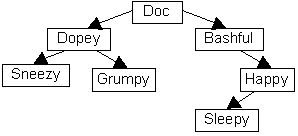Tree Vocabulary

• Vocabulary. Use the diagram of the hierarchical tree shown on page 401 for the following:
1. Which node is the root of the tree?
2. How many leaves on this tree?
3. How many nodes on level 2?
4. What is the height(or depth) of the tree?
5. How many children does "President" have?
6. Is this a binary tree?
7. Is the tree with root "VP Sales/Market" a binary tree?
8. "Dir. Software" is the parent of what node?
9. Name the ancestors of "Product Mgr".

• Shape. Draw a binary tree with 4 levels so that each node (except the last level) has two children.
1. How many nodes does the tree contain?
2. What is the smallest number of levels required to store 10,000 nodes in a binary tree?
3. What advantage is there to organizing data in shallow trees such as these?
4. What is the smallest and the largest possible number of leaves in a binary tree containing exactly six non-leaf nodes?

• Recursion. Why is a tree an inherently recursive structure?
1. What does it mean to traverse a tree?
2. If you follow the Traverse pseudocode on page 404, in what order might you list the names in this tree?• Applications. Trees are often used for representing branching structures or processes such as   ?

Continue to:  Unit 8 / Prev / Next